Published: 25 June 2019

# The results of calculated and experimental determination of the frequency response of a mechanical system with gaps

Mikhail Leontiev1
Dmitry Nasonov2
Anzhelika Volkhonskaya4
1, 3, 4Bauman State Technical University (Kaluga Branch), Kaluga, Russian Federation
2Mechanical Engineering Research Institute of the Russian Academy of Sciences, Moscow, Russian Federation
Corresponding Author:
Dmitry Nasonov
Views 33

#### Abstract

For the first time, an explanation of the practical differences between the results of the calculated and experimental determination of the frequency response of a mechanical system with gaps is proposed in this paper.

## 1. Introduction

The extensive experimental and calculated studies carried out before have significantly reduced the static and dynamic loading of marine planetary gears [1-6] etc.

At the same time, as it always happens in the course of such works, the researchers came across the cases of incomplete mutual compliance of the results of the calculation and the experiment. From the point of view of further improvement of the calculated and experimental models and methods just these cases are of the greatest interest. One of them, connected with the definition of the amplitude phase frequency characteristics (APFC) of a gearbox housing parts, is discussed below.

## 2. The main problem

The examples of experimental  and calculated  dynamic characteristics of housing parts of a marine gearbox are shown in Fig. 1 and 2. They represent the modules and phases of the frequency characteristics of ${H}_{i-j}$, which are complex frequency-dependent relations of vibration acceleration of the object in point $i$ to the force that caused this acceleration, applied in point $j$:

${H}_{i-j}=\frac{{a}_{i}\left(\omega \right)}{{F}_{j}\left(\omega \right)}.$

Experimental characteristics, presented at Fig. 1 were obtained with using the methods and tools described in , at points on the housing of a full-scale marine gearbox, hung on the lifting mechanism in the shop of the manufacturer.

The presented calculated characteristics were obtained by using the finite element method on models constructed by means of the ANSYS software complex . It can be seen at Figs. 1 and 2, that the presented calculated and experimental amplitude phase frequency characteristics, have significant differences between them, although they are similar in levels and form.

First of all, it concerns the fact that the calculated curves are smoother.

Fig. 1The amplitude phase frequency characteristic of the response at point “20” to the excitation at point “2”: a) design (calculated) characteristics , b) experimental characteristics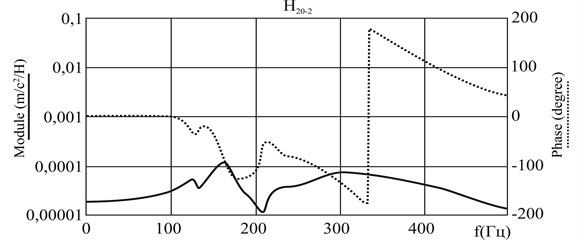a)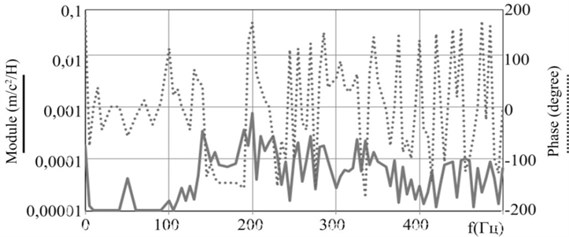b)

## 3. The analysis of the revealed discrepancies

The experience of the study of dynamic characteristics (responses) shows  etc., that the presence of a large number of “bursts” on the amplitude curve together with the “chaotic” behaviour of the phase curve demonstrates the presence of “noises”, which has a sufficient influence on the resulting response of the APFC system.

It is true for the calculated characteristics, when the level of the dynamic response of the system, determined at some control point becomes commensurate with the level of accumulated calculation errors, or, as it is shown below, when the computational model does not adequately describe the object of study.

For experimental curves, this may be a consequence of both, for example, “noises” in the path of response registration or incorrect signal processing, and the features of the studied object, that will be discussed below.

At Fig. 2(a), (b) there are two amplitude phase frequency characteristics ${H}_{5-6}$ and ${H}_{6-5}$ obtained in the course of the experiments . In fact, these are the results of two independent experiments: the excitation at point “6”, the response at point “5” and the excitation at point “5”, the response at point “6”. According to the property of the displacement reciprocity, these frequency responses should be identical. However, at Fig. 2. it can be seen, that they are not identical, although similar.

In addition, the phase characteristics of functions ${H}_{5-6}$ and ${H}_{6-5}$ shown at Fig. 2(a), (b) are not smooth, as well as at Fig. 1(b). It was noted above that it can testify, in particular, to errors while obtaining and processing experimental data. Let us analyze this hypothesis.

According to the work , the main attention in obtaining experimental data was paid to the verification of their reliability. It was conducted according to two criteria. One of them made it possible to estimate the frequency range in which the results of the taken measurements were reliable, with the use of the phase spectra of the input dynamic characteristics (${H}_{1-1}$, ${H}_{2-2}$, ..., ${H}_{20-20}$). Another one confirmed the correctness of the measurement of the modules of the obtained frequency characteristics.

Fig. 2Experimental  APFC responses at points “5” a) and b) “6” for the excitation at points “6” (H5-6) and “5” (H6-5); the calculated  APFC response at point “6” to the, excitation at point “5” c)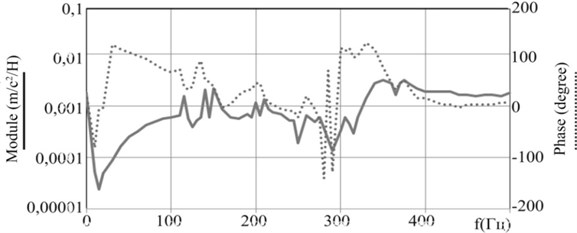a)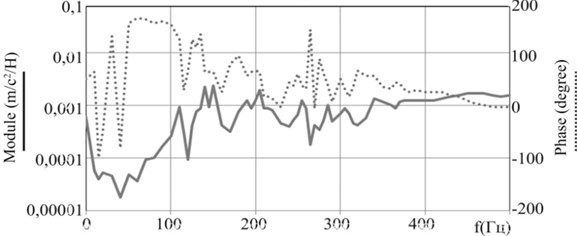b)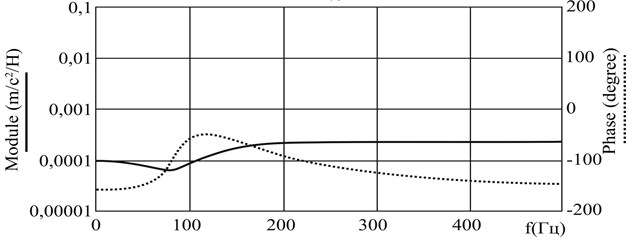c)

The first criterion was based on the fact that the sign of the phase of the input dynamic characteristic (${H}_{i-j}$, $i=j$) should remain constant throughout the whole frequency range, due to the unidirectionality of the vibrational energy flow at the point of its determination. (The energy is transmitted from the vibration exciter to the object of the study, and not vice versa). Applying the conclusions of the work  based on what has been said above, to the experimental characteristics shown at Fig. 1 and 2, one can obtain that these characteristics are reliable in the frequency range 20-220 and 330-420 Hz.

It follows from Fig. 2(a), (b), that though the characteristic ${H}_{6-5}$ has more pronounced responses, but shapes of amplitude frequency characteristics (AFC) ${H}_{5-6}$ and ${H}_{6-5}$ in these ranges match more than in the range of 0-20 Hz and 220-330 Hz, where the differences are more obvious. This indirectly confirms the correctness of analyzed experimental data.

The second of the criteria used in the work  was based on Newton's second law. According to this criterion, the error in determining the modules of dynamic characteristics in the course of the experiments did not exceed 5 %.

## 4. The most likely causes of the identified discrepancies

Thus, having analyzed the available experimental data and not revealed any great mistakes during their obtaining, let’s pay attention to other reasons of the discrepancy between calculated and experimental results. As it was mentioned already, such reasons may be a consequence of not quite adequate description of some of the features of the design of the object of study by its the computational model, namely, the gaps in kinematic pairs.

The satellite node model used in the work  to obtain the calculated APFC (Figs. 1(a) and 2(c)), and built in accordance with the standard approach applicable in the ANSYS software package for modelling such nodes, is schematically shown at Fig. 3.

Fig. 3The design schemes of a satellite node of the 1st stage when calculating APFC: a)  during the experiment, b)  on the working mode (in): 1 – the body of the carrier; 2 – a bogus element; 3 – an axis of the satellite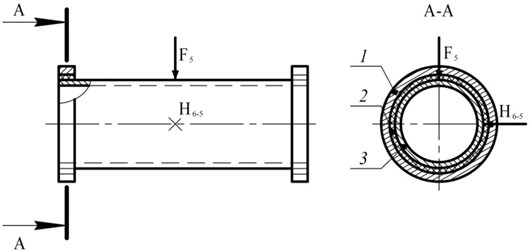a)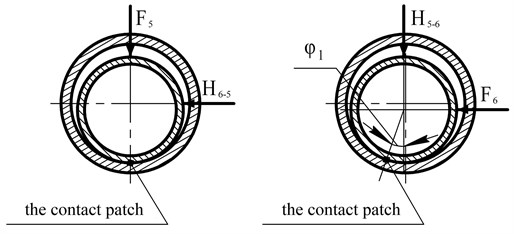b)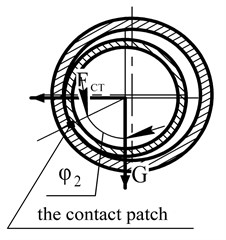c)

At Fig. 3(b) the schemes of the same satellite node are presented during the experimental determination of the frequency characteristics of ${H}_{5-6}$ (the excitation is at point “6”, the response control is at point “5”) and ${H}_{6-5}$ (the excitation is at point “5”, the response control is at point “6”). They obviously show the difference between these two experiments in terms of the location of the contact points of the satellite axis and the planet carrier cheek (zone of maximum stiffness in the joining) relatively to the direction of the vibration excitation action.

At Fig. 3(c) there is a design scheme of a satellite note at the moment of the operation of the gearbox. The contacts in the joining of the satellite axis and the carrier are located in the direction of the resultant force, from the static load transmitted by the satellite and the weight of the satellite node, that is different from the first (Fig. 3(a)), and from the second (Fig. 3(b)) cases.

From the mutual comparison of the presented calculated schemes it becomes obvious, that:

– First, the stiffness and damping parameters of the object at the calculated scheme (Fig. 3(a)) mast differ from similar parameters in the diagrams at Fig. 3(b) and Fig. 3(c), which can highly likely explain the identified differences between the calculated and experimental APFC;

– Secondly, the stiffness and damping parameters of the object while determining the frequency characteristics of ${H}_{5-6}$ and ${H}_{6-5}$ are different too, therefore, the characteristics of ${H}_{5-6}$ and ${H}_{6-5}$ can not coincide as this is shown at Fig. 2(a) and Fig. 2(b);

– Third, the stiffness and damping parameters of the object at carried out the experimental study of its dynamic characteristics (Fig. 3(b)) differ from the analogue parameters in case of the gearbox operation (Fig. 3(c)).

Also one should note that the explanation of the fact of more pronounced responses on the characteristic ${H}_{6-5}$, revealed in the comparison of ${H}_{5-6}$ (Fig. 2(a)) and ${H}_{6-5}$ (Fig. 2(b)), follows right from the consideration of the design schemes shown at Fig. 3(b): it is obvious that the stiffness of the satellite node in the direction of the impact application while obtaining the characteristic ${H}_{6-5}$ is significantly higher than while obtaining the characteristic ${H}_{5-6}$.

## 5. Conclusions

In the given paper, the results of experimental and calculated determination of the dynamic characteristics of the elements of the planetary gear design are compared, and one of the most obvious reasons for the revealed discrepancies the presence of gaps in kinematic pairs is considered.

On the basis of the fulfilled analysis, we can make two important practical conclusions:

1) To improve the accuracy of mathematical modeling of dynamic characteristics of mechanical systems with gaps it is necessary to take into account the size and location of the contact patches in the joints, that affects significantly the stiffness and damping parameters of the researched object;

2) To increase the reliability of the experimental determination of the frequency response of mechanical systems with gaps, it is necessary while the experiment to reproduce static loads which act on the object of study during its operation in accordance with the purpose.